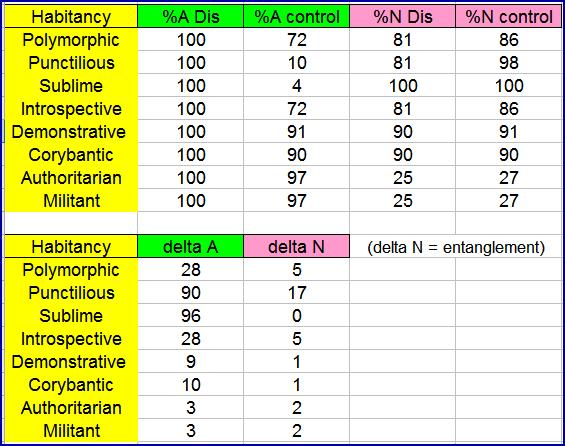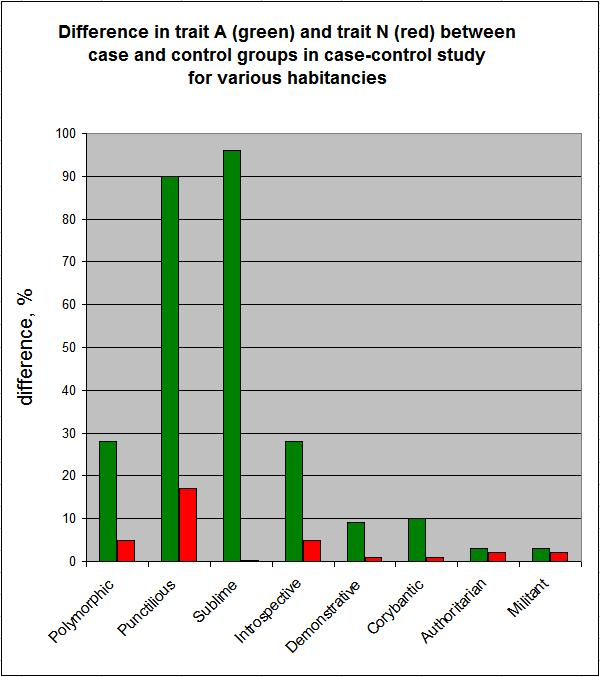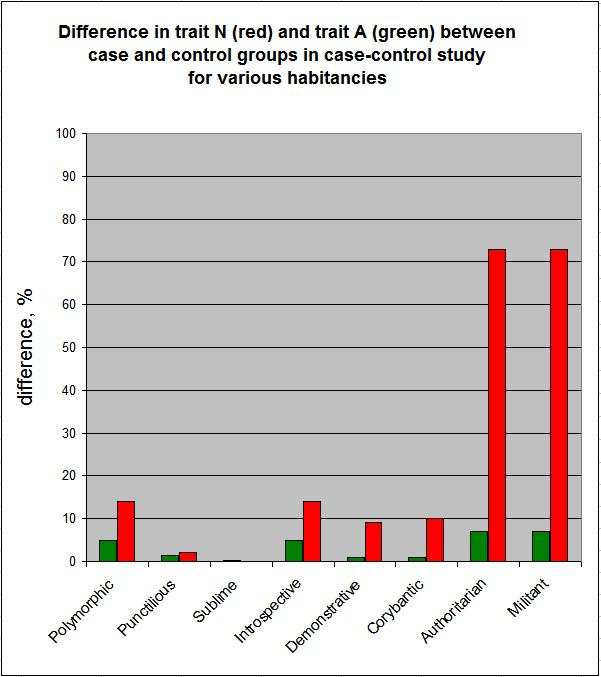NPA personality theory
[ NPA personality theory ]

Entanglement in a case-control study

 [Repeat of post of November 10, 2011] According to the NPA model, the traits N and A are entangled because if one of them is absent, then the other must be present. This is best shown in a quantitative example of what could occur in a case-control study. Let's say that condition or disease "Dis" is causatively related to trait A. That is, trait A must be present for Dis to be phenotypically expressed. Let's say that gene D3 codes for trait A. Let's say that researchers do a case-control study, in which they have a group of Dis subjects and a group of controls from the general population. However, instead of measuring D3 genotypes, they measure gene D2, which codes for trait N. Since traits N and A are entangled, they might get a positive result for association of Dis with gene D2 even though D2 is not causatively involved. In the example below, for different habitancies, we assume that all subjects having condition Dis have a gene related to Dis and also have trait A. We assume that all subjects having trait A have the same random chance of having the gene and condition Dis. We assume that control subjects are drawn randomly from the population. Intuitively one can see that a positive association will be found between condition Dis and gene D2 if there is a partitioning of trait N between the case and control groups. Since Dis is related to trait A, this partitioning can occur if there are more non-sanguine types in the case group than in the control group. Here are the numbers for the various habitancies:(delta = difference between Dis and control group in percent) For example, in a Polymorphic habitancy 100% of the Dis subjects will have trait A compared to 72% of the control group. Similarly, 81% of the Dis subjects will have trait N, compared to 86% of controls. The 5% difference in trait N (by entanglement) should be large enough to be detected in the study. (Actually, the researchers would be tabulating geneotypes of gene D2 rather than a phenotypic trait, but the numbers would be similar if we presented a more complicated version tabulating genotypes related to traits A and N.) Note that sizeable values of N trait entanglement are obtained for the Polymorphic, Punctilious and Introspective habitancies. Note that there is no chance at all of seeing entanglement in the Sublime habitancy, since virtually all of the individuals in both the case and control groups have the N trait. Presenting the above numbers graphically, we obtain:In the figure above, the red bars indicate the entanglement of traits A and N, as reflected in the difference in trait N between the case and control groups. If we assume conversely that condition Dis (say, smiling) is causatively related to trait N, and the entanglement is reflected in the difference in trait A between the case and control groups, then we obtain the figure below:In the figure above, the green bars indicated the entanglement of traits A and N, as reflected in the difference in trait A between the case and control groups. Thus, one can see that due to entanglement a research group doing a case-control study could mistakenly conclude that smiling is (negatively) related to aggression. According to the NPA model, smiling is causatively related to the gene for trait N, and any relation to trait A is "guilt by association." -------------------- (To reply to this post, use the post of 10/XI/11, or start a new post.)